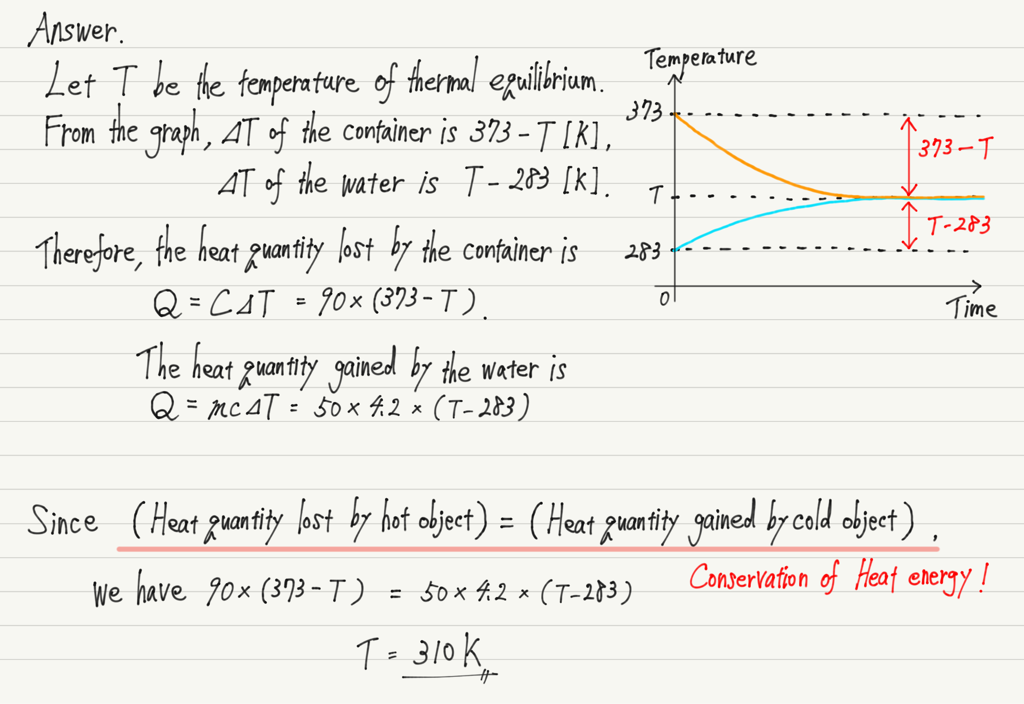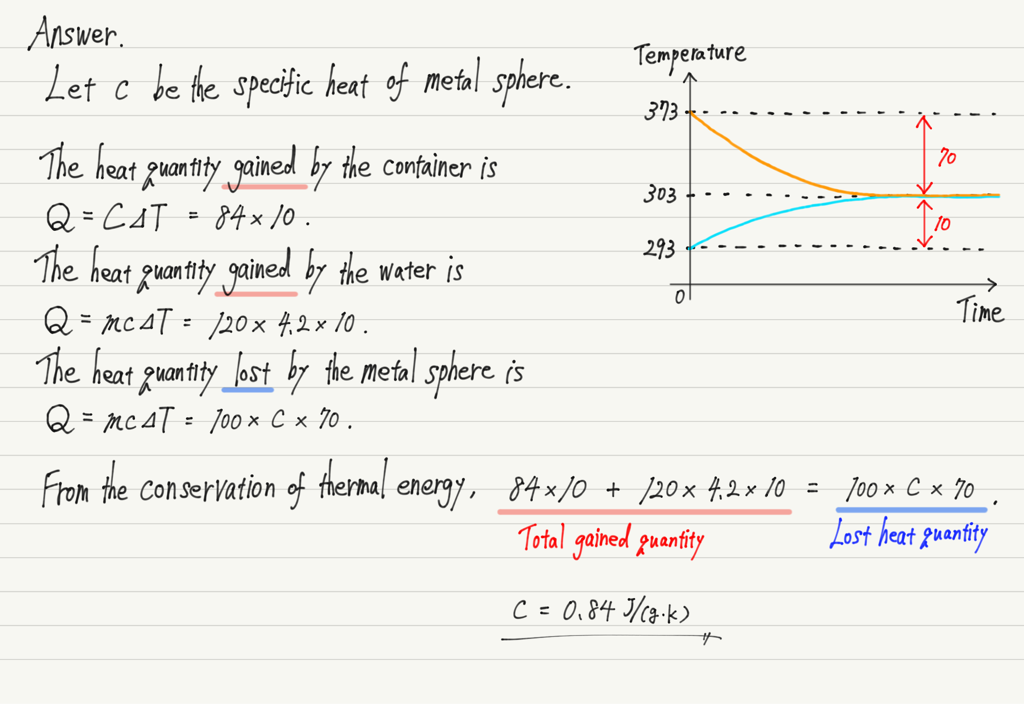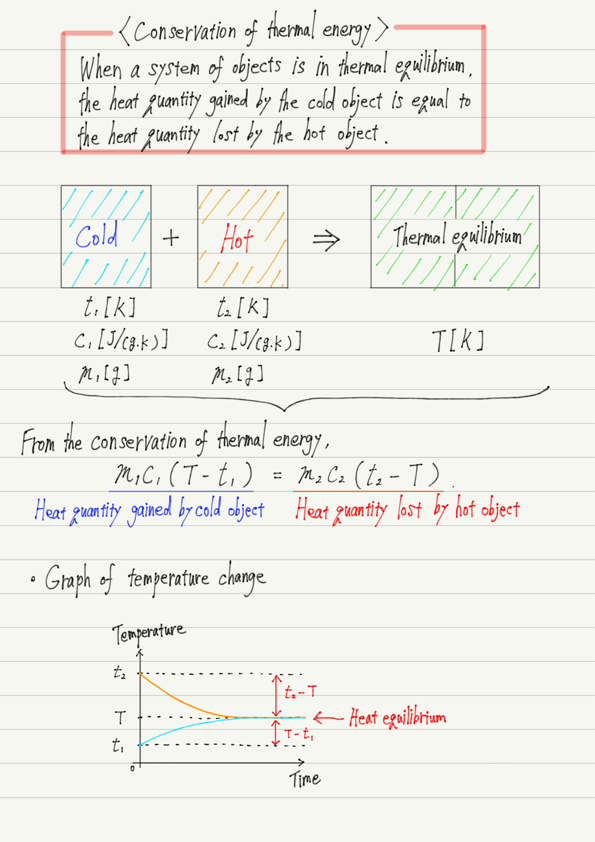# Conservation of Heat Energy

(This article was translated from Japanese to English using DeepL.)

Q. A container heated to 373 K (heat capacity 90 J/K) was filled with 50 g of 283 K water. After a time, the container and the water reached the same temperature. Find that temperature.
The heat exchange is assumed to occur only between the container and the water, and the specific heat of water is 4.2 J/(g・K).

It is a typical question, but many people have difficulty with it.
In this lecture, you will learn how to think about this question.

## Thermal Equilibrium

It may seem confusing with all the numbers in the statement, but let’s first see what is happening before we start the calculation.

When cold water is poured into a hot container, it settles to a certain temperature.

This is the only phenomenon that is occurring.

If objects with different temperatures are placed together, they will eventually reach the same temperature and then their temperatures will stop changing (Thermal equilibrium).

In other words, this question is just that: “Find the temperature when the container and water are in thermal equilibrium.

Now, there is a typical mistake that first-time students often make in this question.

Typical mistake: Since it is between 373 K and 283 K, (373+283)/2=328 K

Indeed, the temperature of thermal equilibrium is between the higher and lower temperatures.
However, it is not always exactly in the middle.
Let’s look at the correct solution.

## Answer and ExplanationThe key to solving this question is the exchange of heat.
Thermal equilibrium occurs when heat is passed from a hot object to a cold object.

Heat is a form of energy, but energy does not just appear and disappear of its own accord.
In other words, all the heat emitted by the hot object is received by the cold object.

The aforementioned “Typical mistake” occurred because the specific heat of the object was not taken into account.
The same heat quantity is not passed and received, but the increase or decrease in temperature is not the same, so the temperature will not be in the middle.

## Exercise

Finally, let’s do three object patterns.

Q. A container with a heat capacity of 84 J/K contained 120 g of water and the temperature was constant at 293 K.
When a 100 g metal sphere heated to 373 K is placed in it, the total temperature becomes 303 K.
Find the specific heat of this metal sphere.
The heat exchange is assumed to occur only between the container and the water, and the specific heat of water is 4.2 J/(g・K).

Even if the number of objects increases, it can be solved in the same way by focusing on “which object loses heat and which gains heat”.

Note that the specific heat is different for each substance, so this calculation will give you an approximate idea of what metal the sphere is made of.

The answer is as follows.## Summary of this lecture## Next Time

Next, we will study phase transitions of matter.

https://www.yukimura-physics.com/entry/therm-f06

error: Content is protected !!